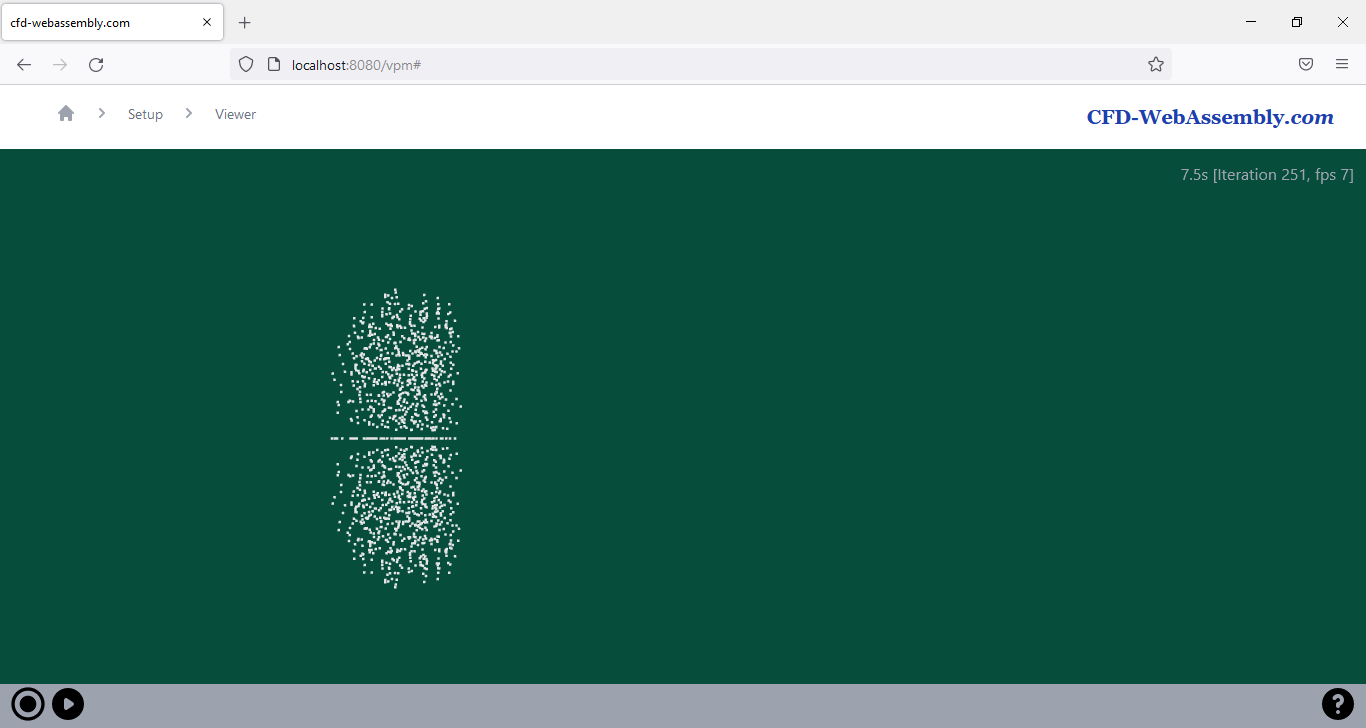# Rust WebAssembly — Sharing data between WebWorkersBenchmark prior to implementation — 1000 vortons with locally hosted webserver

# Serialization

`pub fn to_json(&self) -> JsValue {        JsValue::from_serde(&self.simulation).unwrap()    }pub fn to_array_buffer(&self) -> ArrayBuffer {        let b = bincode::serialize(&self.simulation).unwrap();        Uint8Array::from(&b[..]).buffer()    }pub fn to_shared_array_buffer(&self) -> SharedArrayBuffer {        let b = bincode::serialize(&self.simulation).unwrap();        let mut r = SharedArrayBuffer::new(b.len() as u32);        let mut a = Uint8Array::new(&r);        for i in 0..b.len() { a.set_index(i as u32, b[i]); }        r    }`
`pub fn from_json(content: JsValue) -> Result<Solver, JsValue> {        match JsValue::into_serde(&content) {            Ok(sim) => Ok(Solver { simulation: sim} ),            Err(e) => Err(JsValue::from_str(format!("Unable to parse to simulation. Error {}", e).as_str())),        }    }pub fn from_array_buffer(content: ArrayBuffer) -> Result<Solver, JsValue> {        let a = Uint8Array::new(&content);        match bincode::deserialize(&a.to_vec()[..]) {            Ok(sim) => Ok(Solver { simulation: sim } ),            Err(e) => Err(JsValue::from_str(format!("Unable to retrieve simulation from ArrayBuffer. Error {}", e).as_str())),        }    }pub fn from_shared_array_buffer(content: SharedArrayBuffer) -> Result<Solver, JsValue> {        let a = Uint8Array::new(&content);        match bincode::deserialize(&a.to_vec()[..]) {            Ok(sim) => Ok(Solver { simulation: sim } ),            Err(e) => Err(JsValue::from_str(format!("Unable to retrieve simulation from SharedArrayBuffer. Error {}", e).as_str())),        }    }`
`switch (use_simulation_format) {      case 0: // json        self.postMessage({ on_simulation: true, simulation: wasm_solver.to_json() });        break;      case 1: // array buffer        self.postMessage({ on_simulation_array_buffer: true, simulation: wasm_solver.to_array_buffer() });        break;      case 2: // shared array buffer        self.postMessage({ on_simulation_shared_array_buffer: true, simulation: wasm_solver.to_shared_array_buffer() });        break;      default: // Default: array buffer        self.postMessage({ on_simulation_array_buffer: true, simulation: wasm_solver.to_array_buffer() });    }`